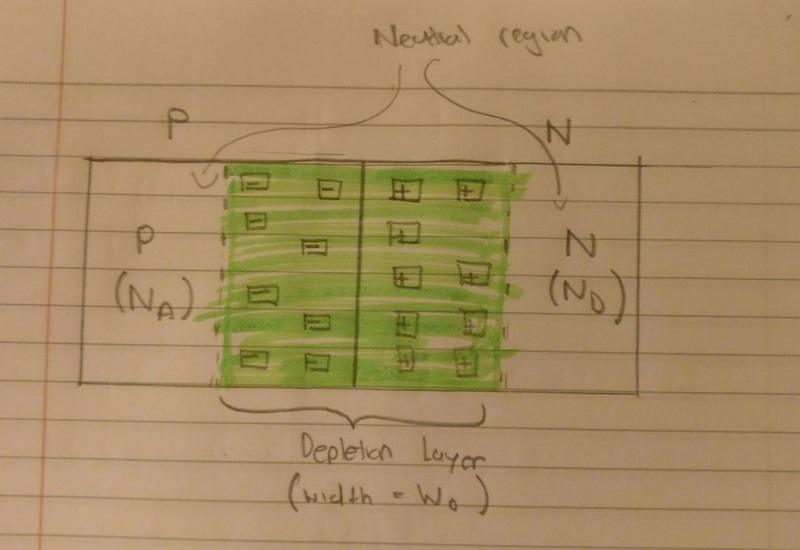# PN Junction Depletion Layer

• CoolDude420
In summary, the P and N regions are separated by a PN junction. At 300K as the acceptor atoms on the P side and donor atoms on the N side are ionised, there is a very high concentration of holes on the P side and electrons on the N side. Diffusion occurs. Holes go to N side. Electrons go to P side. As the holes go to the N side they leave behind negatively charged acceptor atoms. And the electrons from the N side leave behind positvely charged donor atoms. So at this point there is a large concentration of negatively charged acceptor atoms near the junction and a large conc. of positvely charged donor atoms near the junction. This area is known as the depletion

## Homework Statement

I'm doing a course in solid state electronics and we just started PN junctions etc. I'm a bit confused.
So here is what I know so far:

Initially the P and N regions are separated by a a PN junction. At 300K as the acceptor atoms on the P side and donor atoms on the N side are ionised, there is a very high concentration of holes on the P side and electrons on the N side.
Diffusion occurs. Holes go to N side. Electrons go to P side. As the holes go to the N side they leave behind negatively charged acceptor atoms. And the electrons from the N side leave behind positvely charged donor atoms. So at this point there is a large concentration of negatively charged acceptor atoms near the junction and a large conc. of positvely charged donor atoms near the junction. This area is known as the depletion layer.

Now here is my question relating to this diagram:In my notes it says that concentration of acceptor atoms in the neutral region of the P side(i.e excl the depletion layer) is Na. However that's clearly a contradiction because some of those Na acceptor atoms are now in the depletion layer. So shouldn't Na be the total number of acceptor atoms in the entire P region including the part of the depletion layer which is in the P side. Same for N side.

## The Attempt at a Solution

The donor and acceptor atoms don't move. They are fixed in the lattice. Only the mobile charges, the holes and electrons, can move. Also note that the Na and Nd quantities are charge densities - i.e, so many atoms per cubic centimeter. So there are Na acceptor atoms per cubic centimeter everywhere on the P side, but in the neutral region they are neutralized by Na holes per cubic centimeter, so that region is charge neutral. In the depletion region, the acceptor charge is "uncovered" because the holes have moved out, so all that remains is the fixed lattice charge of Na acceptor atoms per cubic centimeter. Does this help?

•CoolDude420
phyzguy said:
The donor and acceptor atoms don't move. They are fixed in the lattice. Only the mobile charges, the holes and electrons, can move. Also note that the Na and Nd quantities are charge densities - i.e, so many atoms per cubic centimeter. So there are Na acceptor atoms per cubic centimeter everywhere on the P side, but in the neutral region they are neutralized by Na holes per cubic centimeter, so that region is charge neutral. In the depletion region, the acceptor charge is "uncovered" because the holes have moved out, so all that remains is the fixed lattice charge of Na acceptor atoms per cubic centimeter. Does this help?

Amazing. Thank you for the brilliant explanation!